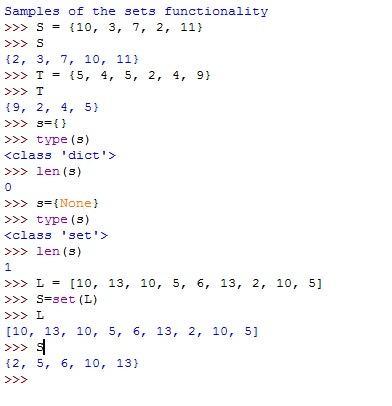# Sets in Python

Python sets: Here, we are going to learn about the sets in Python with various types of operations and examples.
Submitted by Yash Khandelwal, on April 06, 2019

Python provides the functionality of the mathematical set. As in Mathematical sets, we use curly braces ({}) in Python to declare a set we use these brackets. These curly braces in python denote the set.

As we know that dictionary also uses curly braces but the basic difference in sets and the dictionaries is that In dictionary literals by the fact that all the items in a dictionary are connected through a colon(:) I.e the key and the value or we can say the key-value pairs. While sets only contain a simple value.

As we know that lists are ordered it depends on the order of the elements i.e the values at what position they are is fixed until you perform the operations in the list.

But sets are unordered in nature every time you print the set you will see the random arrangements of the elements. Because of that, we cannot access the elements of a set at a particular location. And also set does not support the duplicates as all the elements in a set occurred only at once.

There is one important difference that Python set is having with mathematics: In python, the expression {} does not represent the empty set. In order to use the curly braces for a set, the set must contain at least one element.

The following sequence demonstrates these sets properties. These all properties are all same; like in mathematics.

Note: The expression set() produces a set with no elements, and thus represents the empty set. Python reserves the {} notation for empty dictionaries.There are many operations we can perform on sets likewise in mathematical sets,

Operation Mathematical Notation Python Syntax Type Description/Meaning
Union A∪B A | B Set Elements in A or B or both
Intersection A∩B A&B Set Elements common to both A and B
Set Difference A-B A-B Set Elements in Abut not in B
Symmetric Difference A⊕B A ˆ B Set Elements in A or B , But not in both
Set Membership x ∈ A x in A Boolean X is a member of A
Set Membership x ∈/ A x not in A Boolean X is not a member of A

Note: You can use add(), delete(), remove(), discard() like functions in set also same as likewise in lists etc.

Example:

```# Code for Sets in python
print("Samples of the operations performed by sets")

S = {2, 5, 7, 8, 9, 12}
T = {1, 5, 6, 7, 11, 12}

print("Union:",S|T)
print("Intersection:",S&T)
print("Set Difference:",S-T)
print("Symmetic Difference:",S^T)
print("Set Membership:", 8 in S)
print("Set Membership:", 8 not in S)

```

Output

```Samples of the operations performed by sets
Union: {1, 2, 5, 6, 7, 8, 9, 11, 12}
Intersection: {12, 5, 7}
Set Difference: {8, 9, 2}
Symmetic Difference: {1, 2, 6, 8, 9, 11}
Set Membership: True
Set Membership: False
```

### Python Frozenset

frozenset is the class in the set which once assigned can not be changed again i.e. they are immutable in nature.As we know that set are mutable.But the frozen sets are immutable.

We know that the tuple is the immutable lists like same the Frozen set in the immutable set.

So The operations we perform in set is not supported in the Frozenset like union(), intersection(), difference(), symmetric_difference() etc.

Note: Sets are mutable and unhashable in nature, so we cannot use them as dictionary keys. But The frozensets are hashable and can be used as keys to the dictionary.

Preparation